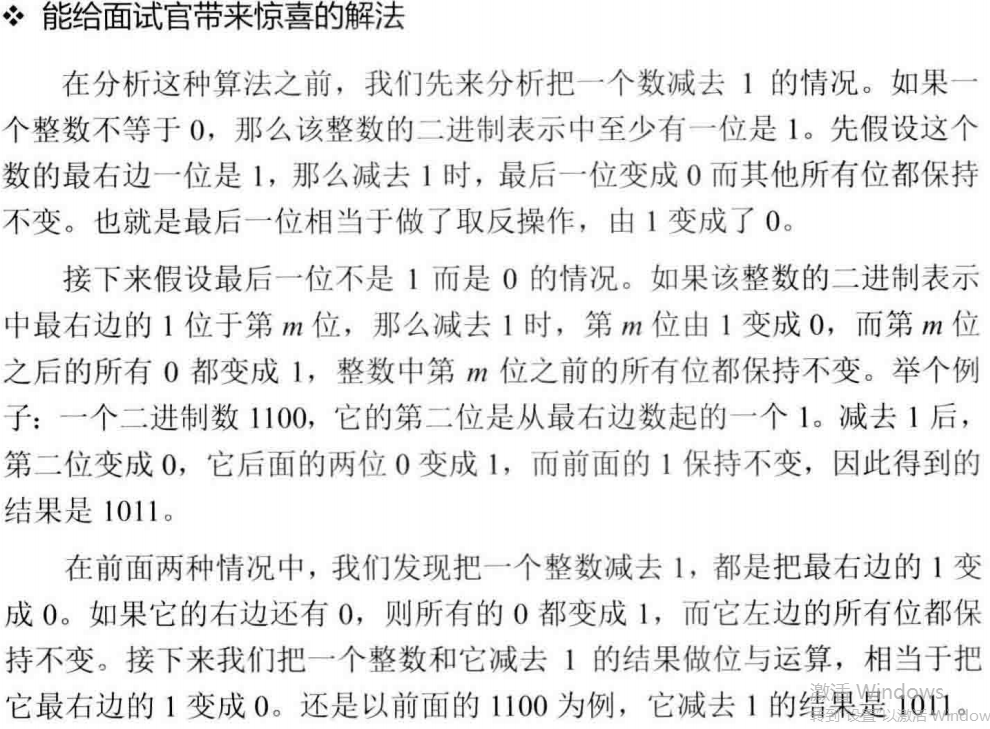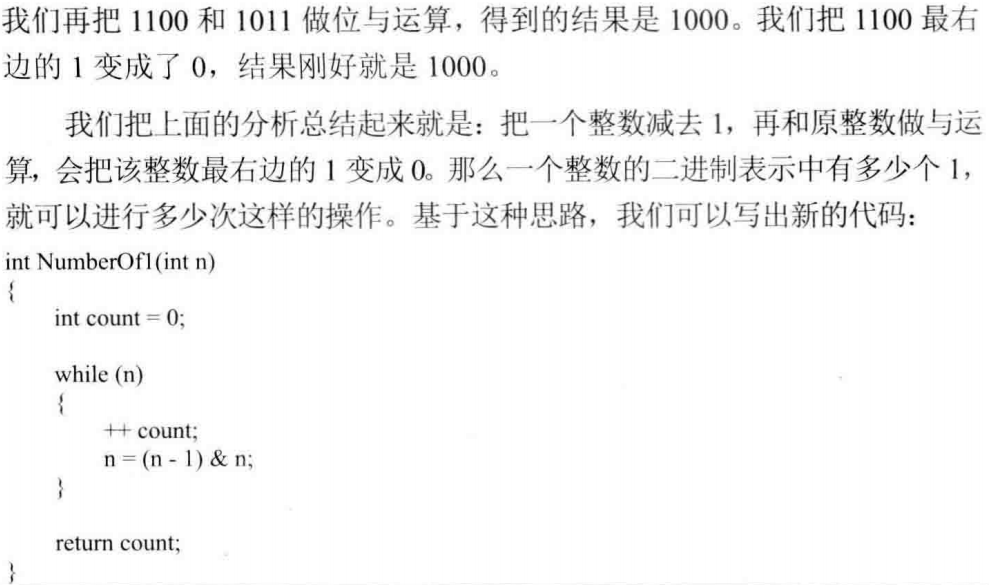法一：分析

`00001010<<2 = 00101000`

`00001010>>2 = 00000010`

代码

```function NumberOf1(n)
{
let flag = 1;
let count = 0;
while(flag){
if(flag & n){
count++;
}
flag = flag << 1;
}
return count;
}
```

法二：分析0条评论